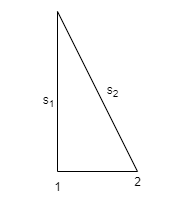# Problem: Two identical loudspeakers, speaker 1 and speaker 2, are 2.0 apart and are emitting 1700- sound waves into a room where the speed of sound is 340 . Is the point 4.0 in front of speaker 1, perpendicular to the plane of the speakers, a point of maximum constructive interference, a point of perfect destructive interference, or something in between?

###### FREE Expert Solution

Constructive interference:

$\overline{){\mathbf{∆}}{\mathbf{x}}{\mathbf{=}}{\mathbf{m}}{\mathbf{\lambda }}}$

Destructive interference:

Speed, frequeny and wavelength relationship:

$\overline{){\mathbf{v}}{\mathbf{=}}{\mathbf{f}}{\mathbf{\lambda }}}$From Pythagoras theorem:

s2 = (22 + 42)(1/2) = 4.472###### Problem Details

Two identical loudspeakers, speaker 1 and speaker 2, are 2.0 apart and are emitting 1700- sound waves into a room where the speed of sound is 340 . Is the point 4.0 in front of speaker 1, perpendicular to the plane of the speakers, a point of maximum constructive interference, a point of perfect destructive interference, or something in between?